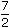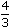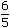# Aptitude - Partnership

1.
A and B invest in a business in the ratio 3 : 2. If 5% of the total profit goes to charity and A's share is Rs. 855, the total profit is:
Rs. 1425
Rs. 1500
Rs. 1537.50
Rs. 1576
Explanation:

Let the total profit be Rs. 100.

 After paying to charity, A's share = Rs.95 x 3= Rs. 57. 5

If A's share is Rs. 57, total profit = Rs. 100.

 If A's share Rs. 855, total profit =100 x 855= 1500. 57

2.
A, B and C jointly thought of engaging themselves in a business venture. It was agreed that A would invest Rs. 6500 for 6 months, B, Rs. 8400 for 5 months and C, Rs. 10,000 for 3 months. A wants to be the working member for which, he was to receive 5% of the profits. The profit earned was Rs. 7400. Calculate the share of B in the profit.
Rs. 1900
Rs. 2660
Rs. 2800
Rs. 2840
Explanation:

For managing, A received = 5% of Rs. 7400 = Rs. 370.

Balance = Rs. (7400 - 370) = Rs. 7030.

Ratio of their investments = (6500 x 6) : (8400 x 5) : (10000 x 3)

= 39000 : 42000 : 30000

= 13 : 14 : 10B's share = Rs.7030 x 14= Rs. 2660. 37

3.
A, B and C enter into a partnership in the ratio::. After 4 months, A increases his share 50%. If the total profit at the end of one year be Rs. 21,600, then B's share in the profit is:
Rs. 2100
Rs. 2400
Rs. 3600
Rs. 4000
Explanation:

 Ratio of initial investments =7 : 4 : 6= 105 : 40 : 36. 2 3 5

Let the initial investments be 105x, 40x and 36x.A : B : C =105x x 4 + 150 x 105x x 8: (40x x 12) : (36x x 12) 100

= 1680x : 480x : 432x = 35 : 10 : 9.

 Hence, B's share = Rs.21600 x 10= Rs. 4000. 54

4.
A, B, C subscribe Rs. 50,000 for a business. A subscribes Rs. 4000 more than B and B Rs. 5000 more than C. Out of a total profit of Rs. 35,000, A receives:
Rs. 8400
Rs. 11,900
Rs. 13,600
Rs. 14,700
Explanation:

Let C = x.

Then, B = x + 5000 and A = x + 5000 + 4000 = x + 9000.

So, x + x + 5000 + x + 9000 = 500003x = 36000x = 12000

A : B : C = 21000 : 17000 : 12000 = 21 : 17 : 12.A's share = Rs.35000 x 21= Rs. 14,700. 50

5.
Three partners shared the profit in a business in the ratio 5 : 7 : 8. They had partnered for 14 months, 8 months and 7 months respectively. What was the ratio of their investments?
5 : 7 : 8
20 : 49 : 64
38 : 28 : 21
None of these
 Now, 14x = 598x = 40yy = 49 x 8y 7 20
 And, 14x = 5112x = 35zz = 112 x = 16 x. 7z 8 35 5x : y : z = x : 49 x : 16 x = 20 : 49 : 64. 20 5